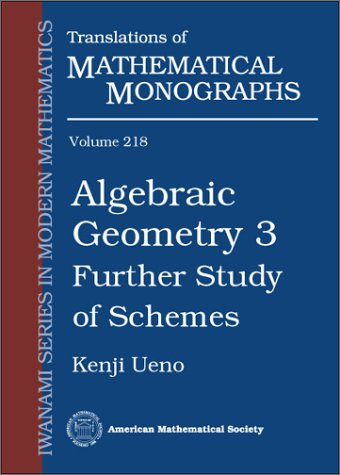## Series Monográficas: Translation of mathematical monographsVol. 47. Nevelson, Nikhail Borisovich; Has'minskii, Rafail Zalmanovich
Stochastic approximation and recursive estimation
QA274 N48

Linear and quasi-linear equations of parabolic type
QA374 L27

Vol. 46. Kurpel', Nikolai Stepanovich
Projection-iterative methods for solution of operator equations
QA329 K86

Vol. 22. Vilenkin, Naum IAkovlevich
Special functions and the theory of group representations
QA351 V54

Vol. 45. Suprunenko, Dmitrii Alekseevich
Matrix groups
QA171 S79

Vol. 21. Shanin, Nikolai Aleksandrovich
Constructive real numbers and contructive function spaces
QA300 S42313

Vol. 44. Ronkin, Lev Isaakovich
Introduction to the theory of entire functions of several variables
QA353.E5 R5713

Statistical problems with nuisance parameters
QA277 L54

Vol. 43. Daletskii, Iurii L'vovich; Krein, Mark Grigor'evich
Stability of solutions of differential equations in Banach space
QA371 D255

Vol. 19. Krasnosel'skii, Mark Aleksandrovich
The operator of translation the trajectories of diferential equations
QA372 K732

Vol. 17. Berezanskii, Iurii Makarovich
Expansions in eigenfunctions of selfadjoint operator
QA320 B45

Vol. 40. Zhelobenko, Dmitrii Petrovich
Compact Lie groups and their representations
QA387 Z44

Vol. 16. Liusternik, Lazar Aronovich

QA315 L57513

Vol. 39. Levitan, Boris Moiseevich; Sargsia, Ishuhan Saribekovich
Introduction to spectral theory : Selfad joint ordinary differential operators
QA379 L47

Vol. 15. Aleksandrov, Aleksandr Danilovich; Zalgaller, Viktor A.
Intrinsic geometry of surfaces
QA641 A53

Vol. 38. Shiriaev, Albert Nikolaevich
Statistical sequential analysis : Optimal stopping rules
QA279.7 S445

Vol. 14. Fuks, Boris Abramovich

QA331 F83

Vol. 37. Freiman, G. A.
Foundations of a structural theory of set addition
QA241 F73913

Vol. 13. Hua, Lo-keng
QA246 H83

Vol. 36. Gol'shtein, Evgenii Grigor'evich
Theory of convex programming
T57.4 G6413

Vol. 12. El’sgol’ts, Lev Ernestovich
Qualitative methods in mathematical analysis
QA303 E5

Vol. 35. Pogorelov, Aleksei Vasil'evich
Extrinsic geometry of convex surfaces
QA649 P62

Vol. 11. Kubilius, Jones
Probabilistic methods in the theory of numbers
QA246 K83

Vol. 34. Livshits, Mikhail Samuilovich
Operators, oscillations, waves (open systems)
QA329.2 L5713

Vol. 10. Delone, Boris Nikolaevich; Faddeev, Dimitrii Konstantinovich
The theory of irrationalities of the third degres
QA242 D4

Vol. 33. Neimark, IUrii Isaakovich
Dynamics of nonholonomic systems
QA845 N44

Vol. 9. Suprunanko, Dmitrii Alekseevich
Soluble and nilpotent linear groups
QA171 S8

Vol. 32. Brodskii, Mikhail Samoilovich

QA329.2 B65

Vol. 7. Sobolev, Sergei L'vovich
Applications of functional analysis in mathematical physics
QA401 S61

Vol. 28. Voronovskaia, E. V.
The functional method and its applications
QA331 V69

Vol. 5. Levin, Boris IAkovlevich.
Distribution of zeros of entire functions
QA351 L4

Vol. 27. Rubinshtein, L. I.
The Stefan problem
QC321 R83

The dispersion method in binary additive problems
QA201 L5

Geometric theory of functions of a complex variable
QA331 G62

Vol. 3. Ljapin, E S.
Semigroups
QA171 L5

Mahler's problem in metric number theory
QA247.5 S67

Vol. 2. Akhiezer, Naum Ilich; Krein, M. G.
Some questions in the theory of moments
QA320 A4

Vol. 24. Gohberg, Israel; Krein, Mark Gregorevich
Theory and applications of volterra operators in Hilbert space
QA320 G6313

### ¿Quién está en línea?

Hay 412 invitados y ningún miembro en línea

Go to top